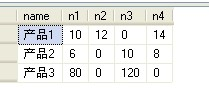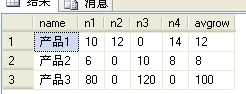# sql 横向求平均值(排除为0项)

1、表名tbl_avgrow2、sql实现语句

select A.*, (A.n1+A.n2+A.n3+A.n4)/B.count1 as avgrow ,B.count1 as countcalc from tbl_avgrow as A left join
(--每行不为零的字段总数
select name ,A1+A2+A3+A4 as count1 from
(
select name,
--筛选
case  when n1=0 then 0 else 1 end A1,
case  when n2=0 then 0 else 1 end A2,
case  when n3=0 then 0 else 1 end A3,
case  when n4=0 then 0 else 1 end A4
from tbl_avgrow
) as counttable
)as B
on A.name =B.name